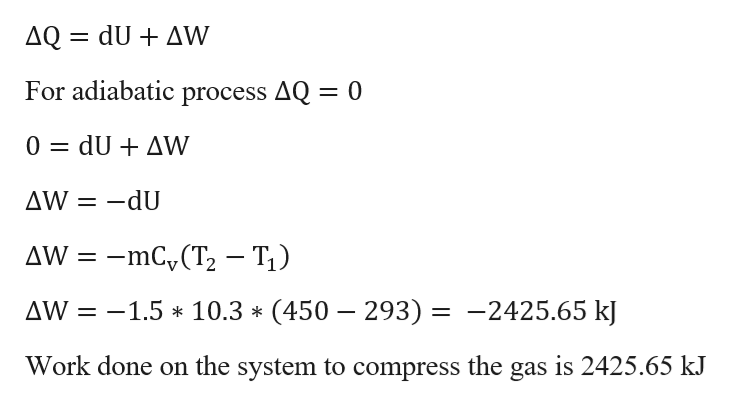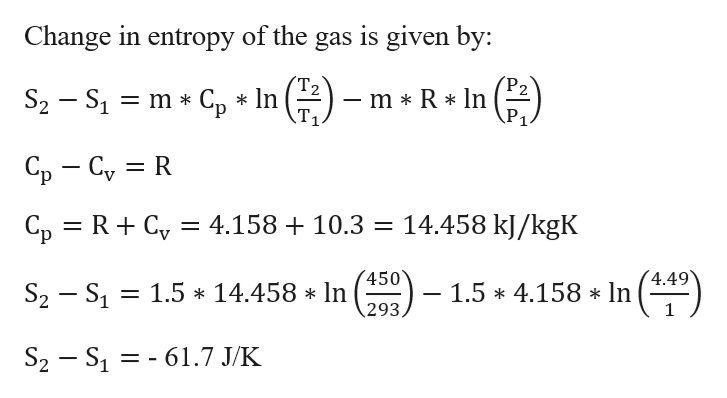A container of 1.5 Kg of gas is at a temperature and pressure of 293 K and 1 bar respectively.   The gas is adiabatically compressed until its temperature and pressure are 450 K, 4.49 bars.  Adiabatic processes are processes with no heat transfer.  The properties of this gas are cv = 10.3 KJ/(Kg K) and R = 4.158 KJ/(Kg K).  Neglect kinetic and potential energy terms.  Use the first law to determine the work into the system.Calculate the entropy production for this process.Is this a reversible process?

Question

A container of 1.5 Kg of gas is at a temperature and pressure of 293 K and 1 bar respectively.   The gas is adiabatically compressed until its temperature and pressure are 450 K, 4.49 bars.  Adiabatic processes are processes with no heat transfer.  The properties of this gas are cv = 10.3 KJ/(Kg K) and R = 4.158 KJ/(Kg K).  Neglect kinetic and potential energy terms.

1. Use the first law to determine the work into the system.
2. Calculate the entropy production for this process.
3. Is this a reversible process?
Step 1

Given Information :

Mass of gas m = 1.5 kg

Initial temperature T1 = 293 K

Initial pressure P1 = 1 Bar = 100 kPa

Final temperature T2 = 450 K

Final Pressure P2 = 4.49 bar = 449 kPa

Assume : The gas is behaving as ideal gas.

Step 2

If kinetic energy and potential energy are neglected, then first law of thermodynamics is as follows.help_outlineImage TranscriptioncloseAQ = dU AW For adiabatic process AQ = 0 dU + ΔW 0 -dU ΔW -mCy (T2 ΔW - T1) = AW = -1.5 10.3 (450 293) = -2425.65 kJ ** Work done on the system to compress the gas is 2425.65 kJ fullscreen
Step 3

Change in entropy of the...help_outlineImage TranscriptioncloseChange in entropy of the gas is given by: (㈜) Т2 S2 S1 m Cp * In m * R In Ср — Су %3D R 14.458 kJ/kgK Cp R C 4.158 10.3 450 S2 S1 1.5 * 14.458 * In 293 - 1.5 * 4.158* In (4.49 61.7 J/K S2 S1 fullscreen

Want to see the full answer?

See Solution

Want to see this answer and more?

Our solutions are written by experts, many with advanced degrees, and available 24/7

See Solution
Tagged in

Mechanical Engineering